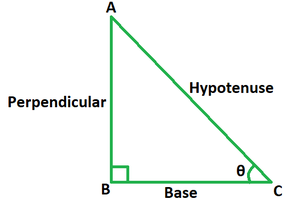Open in App
Not now

• Last Updated : 26 Apr, 2022

Trigonometric identities are equalities using trigonometric functions that hold true for any value of the variables involved, hence defining both sides of the equality. These are equations that relate to various trigonometric functions and are true for every variable value in the domain. The formulae sin(A+B), cos(A-B), and tan(A+B) are some of the sum and difference identities.

### Tangent Trigonometric Ratio

The ratio of any two right triangle sides is called a trigonometric ratio. The tangent ratio is defined as the ratio of the length of the opposite side of an angle divided by the length of the adjacent side.If θ is the angle created by the base and hypotenuse of a right-angled triangle then,

tan θ = Perpendicular/Base = sin θ/ cos θ

Here, perpendicular is the side opposite to the angle and base is the side adjacent to it.

In trigonometry, the tangent addition formula is referred to as the tan(A + B) formula for the compound angle (A+B). It is used when the angle for which the tangent function value is to be determined is supplied as the sum of any two angles. It may alternatively be written as tan(A + B) = sin (A + B)/cos (A + B) since the tangent function is a ratio of the sine and cosine functions.

tan(A + B) = (tan A + tan B)/(1 – tan A tan B)

Derivation

The formula for tangent addition is derived by using the formulas for expansion of sum angle for sine and cosine ratios.

Now, we know that,

tan (A + B) = sin (A + B)/cos (A + B)   …… (1)

Substitute sin (A + B) = sin A cos B + cos A sin B and cos (A + B) = cos A cos B – sin A sin B in the equation (1).

tan (A + B) = (sin A cos B + cos A sin B)/(cos A cos B – sin A sin B)

Dividing the numerator and denominator by cos A cos B, we get

tan (A + B) = [(sin A cos B + cos A sin B)/(cos A cos B)]/[(cos A cos B – sin A sin B)/(cos A cos B)]

tan (A + B) = [(sin A cos B)/(cos A cos B) + (cos A sin B)/(cos A cos B)]/[(cos A cos B)/(cos A cos B) – (sin A sin B)/(cos A cos B)]

tan (A + B) = (tan A + tan B)/(1 – tan A tan B)

This derives the formula for tangent addition of any two angles, A and B.

### Sample Problems

Problem 1. If tan A = 1/2 and tan B = 1/3, find the value of tan (A+B) using the formula.

Solution:

We have, tan A = 1/2 and tan B = 1/3.

Using the formula we get,

tan (A + B) = (tan A + tan B)/(1 – tan A tan B)

= (1/2 + 1/3)/(1 – (1/2)(1/3))

= (5/6)/(1 – 1/6)

= (5/6)/(5/6)

= 1

Problem 2. If tan A = 2/3 and tan B = 4/7, find the value of tan (A+B) using the formula.

Solution:

We have, tan A = 2/3 and tan B = 4/7.

Using the formula we get,

tan (A + B) = (tan A + tan B)/(1 – tan A tan B)

= (2/3 + 4/7)/(1 – (2/3)(4/7))

= (16/21)/(1 – 8/21)

= (16/21)/(13/21)

= 16/13

Problem 3. If tan (A+B) = 15/11 and tan A = 2/11, find the value of tan B using the formula.

Solution:

We have, tan (A+B) = 15/11 and tan A = 2/11.

Let tan B = x.

Using the formula we get,

tan (A + B) = (tan A + tan B)/(1 – tan A tan B)

=> 15/11 = (2/11 + x)/(1 – (2/11)(x))

=> 15/11 = ((2 + 11x)/11)/((11 – 2x)/11)

=> 15/11 = (11x + 2)/(11 – 2x)

=> 165 – 30x = 121x + 22

=> 151x = 143

=> x = 143/151

=> tan B = 143/151

Problem 4. If tan B = 6/13 and tan (A+B) = 9/13, find the value of tan A using the formula.

Solution:

We have, tan (A+B) = 9/13 and tan B = 6/13.

Let tan A = x.

Using the formula we get,

tan (A + B) = (tan A + tan B)/(1 – tan A tan B)

=> 9/13 = (x + 6/13)/(1 – (x)(6/13))

=> 9/13 = ((13x + 6)/13)/((13 – 6x)/13)

=> 9/13 = (13x + 6)/(13 – 6x)

=> 117 – 54x = 169x + 78

=> 223x = 39

=> x = 39/223

=> tan A = 39/223

Problem 5. If sin A = 4/5 and cos B = 5/13, find the value of tan (A+B) using the formula.

Solution:

We have, sin A = 4/5.

It means, cos A = 3/5. So, tan A = 4/3.

Also, cos B = 5/13.

It means, sin B = 12/13. So, tan B = 12/5.

Using the formula we get,

tan (A + B) = (tan A + tan B)/(1 – tan A tan B)

= (4/3 + 12/5)/(1 – (4/3)(12/5))

= (56/15)/(1 – 48/15)

= (56/15)/(-33/15)

= -56/33

My Personal Notes arrow_drop_up
Related Articles